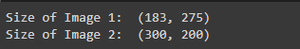Open In App

# Load Images in Tensorflow – Python

For loading Images Using Tenserflow, we use tf.keras.utils.load_img function, which loads the image from a particular provided path in PIL Format. PIL is a Python Imaging Library that gives your Python interpreter access to image processing functions. This library offers a wide range of file format compatibility, a productive internal representation, and somewhat potent image processing features.

The primary image library is built for quick access to information held in a few basic pixel formats. It ought to serve as a strong starting point for a broad image processing tool.

path: Path of the required Image

grayscale: Set true if want to load an Image in grayscale format.

target_size: For loading an image in the required target size. Size format: (Image_height, Image_width)

interpolation: Set for required Interpolation. By default ‘nearest’.

keep_aspect_ratio: Boolean, indicating whether or not to resize photos without distorting their aspect ratio.

Before resizing, the image is cropped in the middle to the desired aspect ratio.

## Python3

 `# Loading the required libraries``import` `tensorflow as tf`` ` `# Defining the path to the image``# which is to be loaded.``# The image_path shall be changed``# according to the requirement.``image_path ``=` `'/content/model_3.png'`` ` `# Calling the load_img function``# from the imported libraries.``# This return an image in PIL``# Format``image_loaded ``=` `tf.keras.utils.load_img(image_path)`` ` `# Printing the obtained image.``image_loaded`

Output:The steps for loading an Image in grayscale are the same as that mentioned above, Just while loading an image we need to set the parameter grayscale = True.

## Python3

 `# Loading the required libraries``import` `tensorflow as tf`` ` `# Defining the path to the image ``# which is to be loaded.``image_path ``=` `'/content/model_3.png'`` ` `# Calling the load_img function from``# the imported libraries. This ``# return an image in PIL``# Format``image_loaded ``=` `tf.keras.utils.load_img(image_path, ``                                       ``grayscale``=``True``)`` ` `# Printing the obtained image.``image_loaded`

Output:In this case, we will load our Image in different target size.

## Python3

 `# Loading the required libraries``import` `tensorflow as tf`` ` `# Defining the path to the image ``# which is to be loaded.``image_path ``=` `'/content/model_3.png'`` ` `# Calling the load_img function from the``# imported libraries. This return an ``# image in PIL Format``image_loaded_1 ``=` `tf.keras.utils.load_img(image_path)`` ` `# Specifying size for the second Image``# Target size = (200,300)``image_loaded_2 ``=` `tf.keras.utils.load_img(image_path,``                                         ``target_size``=``(``200``,``                                                      ``300``))`` ` `# Printing the obtained image.``print``(``"Size of Image 1: "``, image_loaded_1.size)``print``(``"Size of Image 2: "``, image_loaded_2.size)`` ` `image_loaded_2.save(``'/content/out.png'``)`

Output: